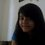# Still confused about integral of ln|sin x|

I don't know why when i'm googling solution for integral of ln|sin x|, the output i clicked always give me the solution integral of log(sin x) but the title is integral of ln|sin x|. Is integral of ln|sin x| equal to integral of log(sin x)?

When i asked the solution to my teacher whom gives that problem, he also confused and make me more confused. He tried many times and always failed.Note by Dina Andini Sri Hardina
6 years, 10 months ago

This discussion board is a place to discuss our Daily Challenges and the math and science related to those challenges. Explanations are more than just a solution — they should explain the steps and thinking strategies that you used to obtain the solution. Comments should further the discussion of math and science.

When posting on Brilliant:

• Use the emojis to react to an explanation, whether you're congratulating a job well done , or just really confused .
• Ask specific questions about the challenge or the steps in somebody's explanation. Well-posed questions can add a lot to the discussion, but posting "I don't understand!" doesn't help anyone.
• Try to contribute something new to the discussion, whether it is an extension, generalization or other idea related to the challenge.

MarkdownAppears as
*italics* or _italics_ italics
**bold** or __bold__ bold
- bulleted- list
• bulleted
• list
1. numbered2. list
1. numbered
2. list
Note: you must add a full line of space before and after lists for them to show up correctly
paragraph 1paragraph 2

paragraph 1

paragraph 2

[example link](https://brilliant.org)example link
> This is a quote
This is a quote
    # I indented these lines
# 4 spaces, and now they show
# up as a code block.

print "hello world"
# I indented these lines
# 4 spaces, and now they show
# up as a code block.

print "hello world"
MathAppears as
Remember to wrap math in $$ ... $$ or $ ... $ to ensure proper formatting.
2 \times 3 $2 \times 3$
2^{34} $2^{34}$
a_{i-1} $a_{i-1}$
\frac{2}{3} $\frac{2}{3}$
\sqrt{2} $\sqrt{2}$
\sum_{i=1}^3 $\sum_{i=1}^3$
\sin \theta $\sin \theta$
\boxed{123} $\boxed{123}$

Sort by:

If you clicked a link to Wolfram Alpha, then what you saw was, in fact, the integral of what you'd asked for. Wolfram Alpha uses $\log$ as $\log_e = \ln$, not $\log_{10}$.

- 6 years, 10 months ago

hey I agree that this integral is very challenging. Firstly, ln|sinx| does not equal log|sinx| nor do their integrals. Secondly, is this a indefinite or definite integral, if so does it have any boundaries?

- 6 years, 10 months ago

If you know Integral Calculus, you may integration by parts.

- 6 years, 10 months ago

but how?

- 6 years, 10 months ago

multinomial in identical distribution

- 6 years, 10 months ago

The indefinite integral of ln lsin xl cannot be expressed in terms of elementary functions. If you have used Wolfram Alpha, you will see that it uses the special function Li(x), so we can't expect that the integral of ln lsin xl can be expressed in terms of functions we usually encounter like ln x, sin x, cos x, and many others.

- 5 years, 7 months ago